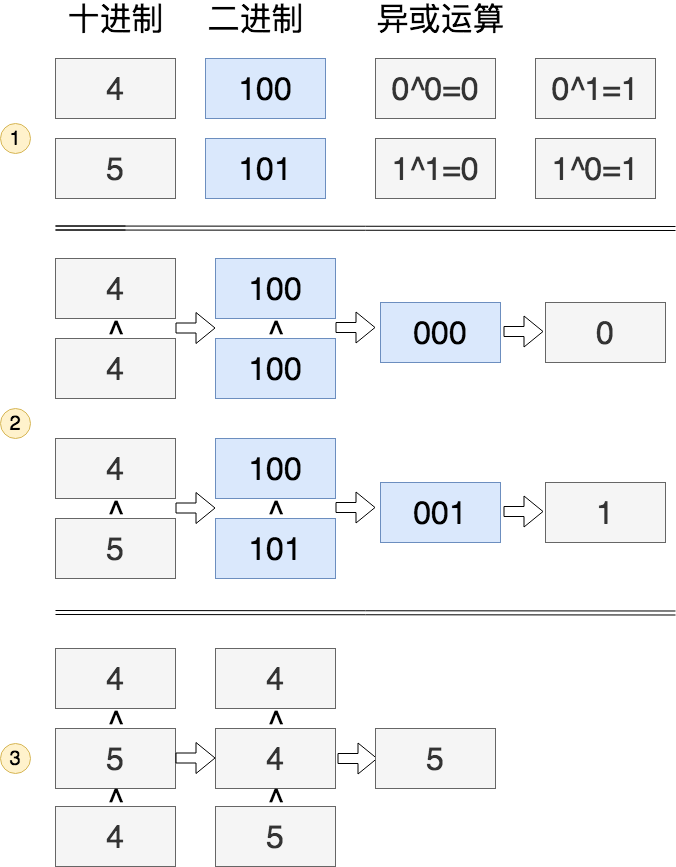# 只出现一次的数字 Single Number

## 题目

Given a non-empty array of integers, every element appears twice except for one. Find that single one.

Note:

Your algorithm should have a linear runtime complexity. Could you implement it without using extra memory?

Example 1:

``````Input: [2,2,1]
Output: 1
``````

Example 2:

``````Input: [4,1,2,1,2]
Output: 4
``````

https://leetcode-cn.com/problems/single-number/

## 思路&题解

``````public int singleNumber(int[] nums) {
HashMap<Integer, Boolean> map = new HashMap<>();
for (int n : nums) {
if (map.containsKey(n)) {
// 第二次出现
map.put(n, true);
} else {
// 第一次出现
map.put(n, false);
}
}
int result = nums;
for (Map.Entry<Integer, Boolean> entry : map.entrySet()) {
if (entry.getValue()) {
continue;
}
result = entry.getKey();
break;
}
return result;
}
``````

``````class Solution {
public int singleNumber(int[] nums) {
int result = 0;
for(int i = 0; i < nums.length; i++) {
result = result ^ nums[i];
}
return result;
}
}
``````

## 知识点分析

### 位的异或运算1. 归零率，相同两个数异或后为零
2. 零和任意数进行异或还是原数字
3. 交换率，两个数异或顺序可以交换

``````void swap(int a, int b) {
a = a ^ b;
b = b ^ a;
a = a ^ b;
}
``````